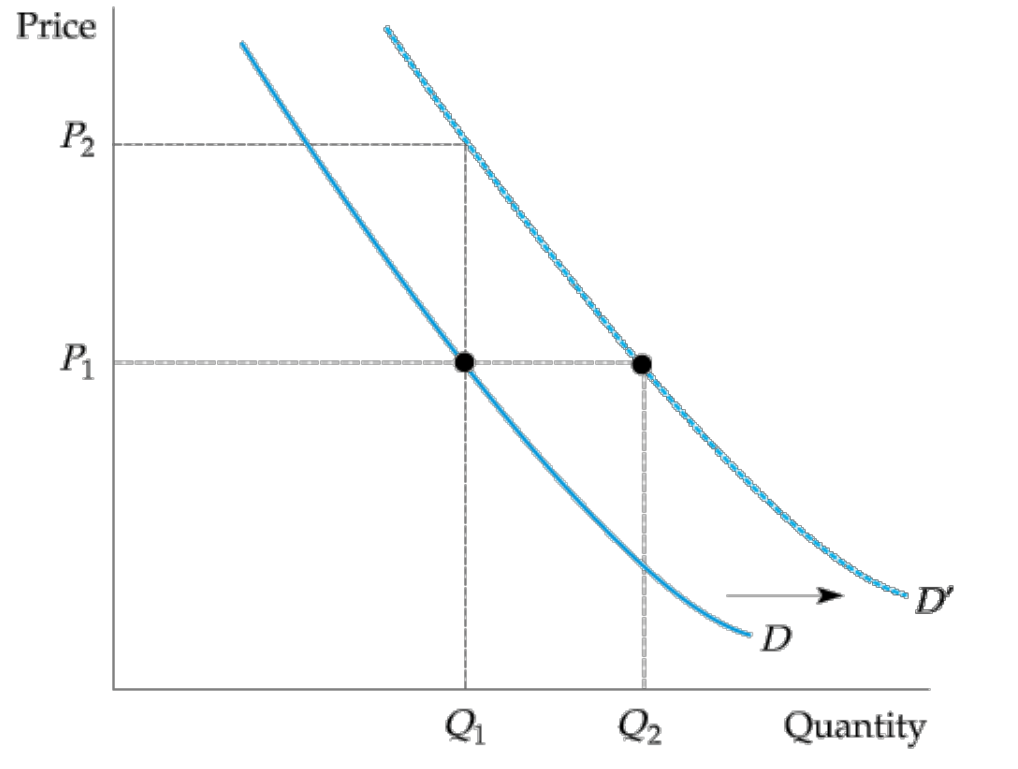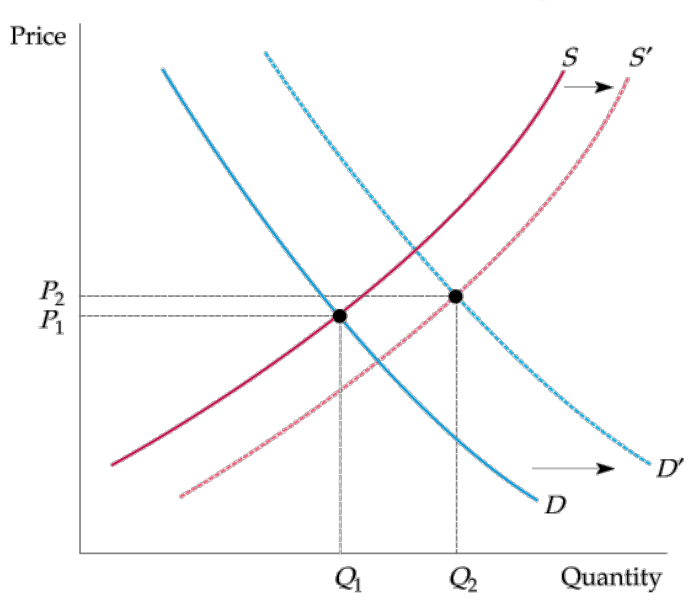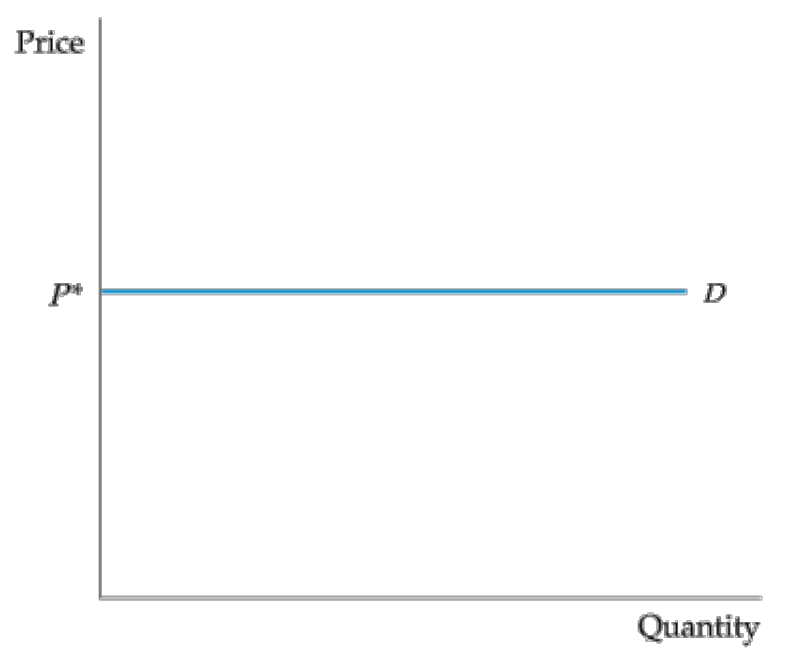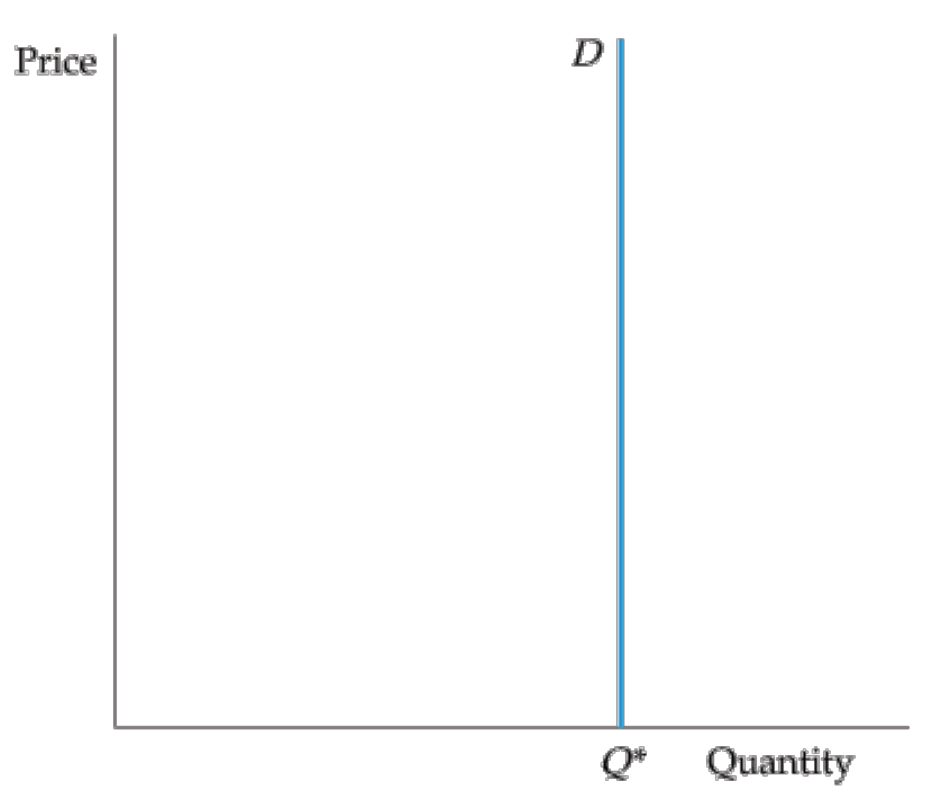# The Basics of Supply and Demand

## Supply and Demand

### The Supply Curve

Q_S=Q_S(P)Higher Price → Larger Quantity (upward)
Lower Production costs → Larger Quantity (that is the right shift of the curve)

### The Demand Curve

Q_D=Q_D(P)• substitutes
• complements

Higher Price → Smaller Quantity (downward)
High income → Larger Quantity (that is the right shift of the curve)

## The Market Mechanism• equilibrium (or market clearing) price: Price that equates the quantity supplied to the quantity demanded.(P_0)
• market mechanism: Tendency in a free market for price to change until the market clears.
• surplus Situation: in which the quantity supplied exceeds the quantity demanded.
• shortage Situation: in which the quantity demanded exceeds the quantity supplied.

This assumption makes sense only if a market is at least roughly competitive. Both sellers and buyers should have little market power (little ability individually to affect the market price)

## Changes in Market Equilibrium## Elasticities of Supply and Demand

elasticity: Percentage change in one variable resulting from a 1-percent increase in another.

• elastic: the change is great (<1)
• inelastic: the change is minor (>1)

E_p=\dfrac{\%\Delta Q}{\%\Delta P} = \dfrac{\frac{\Delta Q}{Q}}{\frac{\Delta P}{P}}=\dfrac{P}{Q}\dfrac{\Delta Q}{\Delta P}

Talking about larger or smaller, we always talk about the absolute value.

• Horizontal Demand CurveInfinite elasticity

• Vertiacal Demand Curvezero elasticity (inelastic)

income elasticity of demand
cross-price elasticity of demand

## Short-Run versus Long-Run Elasticities

Not by a calendar length. The distinctions here are vague.
Short run: fixed cost
Long run: no fixed cost

### Demand

Demand - Price elasticities

• For necessities:
In the short run, necessities have smaller price elasticity of demand;
In the long run, necessities have larger price elasticity of demand.
• For durables:
In the short run, durables have larger price elasticity of demand;
In the long run, durables have smaller price elasticity of demand;

Gasoline belongs to necessities, and automobiles are durables.

### Supply

Secondary supply is therefore less elastic in the long run than in the short run.

## Understanding and Predicting the Effects of Changing Market Conditions

A mathematical problem that is really a piece of cake.